U.S. Department of Transportation
1200 New Jersey Avenue, SE
Washington, DC 20590
202-366-4000

Federal Highway Administration Research and Technology
Coordinating, Developing, and Delivering Highway Transportation InnovationsThis report is an archived publication and may contain dated technical, contact, and link information
 Federal Highway Administration > Publications > Research Publications > Hydraulics Publications > 02078 > 06.Cfm > Bottomless Culvert Scour Study: Phase I Laboratory Report
 Publication Number: FHWA-RD-02-078 Date: November 2003

# Bottomless Culvert Scour Study: Phase I Laboratory Report

### 6. RECOMMENDED PROCEDURES FOR ESTIMATING MAXIMUM SCOUR FOR BOTTOMLESS CULVERTS

#### PROCEDURE USING GKY METHOD FOR REPRESENTATIVE VELOCITY AND SMB EQUATION FOR CRITICAL VELOCITY

The GKY method for representative velocity and the SMB equation for critical velocity with the blocked discharge normalized by the acceleration of gravity (g) and the computed equilibrium depth as the independent regression variable gave the best R2 value, regressing the KADJ (see equation 3) to compute the maximum scour for the laboratory data. The SMB method for computing critical velocity, however, is independent of the flow depth and produces much lower critical velocities than the other methods for fine particle sizes. This will, in turn, result in overly conservative scour estimates for field situations.

The procedure is:

Step 1: Compute representative velocity using: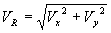(62)

with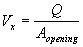(63)

and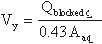(64)

where:

 Vx = velocity in the flow direction, ft/s Vy = velocity orthogonal to the flow direction, ft/s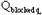= approach flow blocked by the embankment on one side of the channel centerline, ft3/s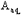= total approach flow area on one side of the channel centerline, ft2 Ablocked = approach flow area that is blocked by the embankments on one side of the channel, ft2

Step 2: Determine critical velocity by applying the SMB equations. The Shields Manning equations can be combined to yield: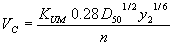(65)

where:

 KUM = 1.0 for SI units or 1.49 for U.S. customary units n = Manning's roughness

Blodgett's equations for average estimates of Manning's n for sand- and gravel-bed channels are: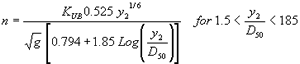(66)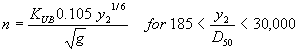(67)

where:

 g = acceleration of gravity= 9.81 m/s2 for SI units or 32.2 ft/s2 for U.S. customary units KUB = 1/1.49 for SI units or 1.0 for U.S. customary units

Step 3: Calculate y2 using: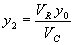(68)

Step 4: Use following regression equation to compute KADJ: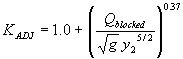(69)

with: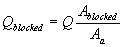(70)

where:

 Qblocked = approach flow blocked by the embankment on one side of the channel centerline, ft3/s Aa = total approach flow area on one side of the channel, ft2 Ablocked = approach flow area that is blocked by the embankments on one side of the channel, ft2

Step 5: Compute maximum scour according to: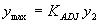(71)

#### PROCEDURE USING MARYLAND DOT (CHANG) METHOD FOR REPRESENTATIVE VELOCITY AND CRITICAL VELOCITY

The recommended procedure is based on using the Maryland DOT (Chang) method for computing both representative velocity and critical velocity. For computing the KADJ factor, the method using the blocked discharge normalized by the acceleration of gravity (g) and the computed equilibrium depth as the independent regression variable was chosen because it is considered to be more applicable to field situations.

The procedure is:

Step 1: Compute representative velocity using the Maryland DOT (Chang) method: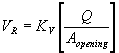(72)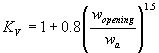(73)

where:

 KV = velocity coefficient to account for flow concentration where side flow converges with main channel flow based on potential flow assumptions Q = total discharge through the culvert, ft3/s Aopening = average flow area within the culvert, ft2 Wopening = average flow width in the culvert, ft wa = width of flow in the approach section, ft

These equations are dimensionally homogeneous and are independent of the system of units as long as they are consistent.

Step 2: Determine critical velocity using the Maryland DOT (Chang) method:

• For D50 > 0.03 m (0.1 ft):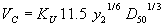(74)

where:

 y2 = equilibrium flow depth, m or ft D50 = sediment size, m or ft KU = 0.55217 for SI units or 1.0 for U.S. customary units
• For 0.03 m (0.1 ft) > D50 > 0.0003 m (0.001 ft):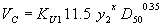(75)

The exponent x is calculated using equation 13: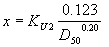(76)

where:

 y2 = equilibrium flow depth, m or ft D50 = sediment size, m or ft KU1 = for SI units or1.0 for U.S. customary units x = exponent from equation 13 KU2 = 0.788 for SI units or1.0 for U.S. customary units
• For 0.0003 m (0.001 ft) > D50: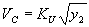(77)

where:

 y2 = equilibrium flow depth, m or ft D50 = sediment size, m or ft KU = 0.55217 for SI units or 1.0 for U.S. customary units

Step 3: Calculate y2 using:(78)

Step 4: Use the following regression equation to compute KADJ: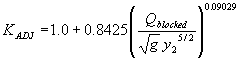(79)

with(80)

where:

 Qblocked = approach flow blocked by the embankment on one side of the channel, ft3/s Aa = total approach flow area on one side of the channel, ft2 Ablocked = approach flow area that is blocked by the embankments on one side of the channel, ft2(81)

Step 5: Compute maximum scour according to: